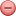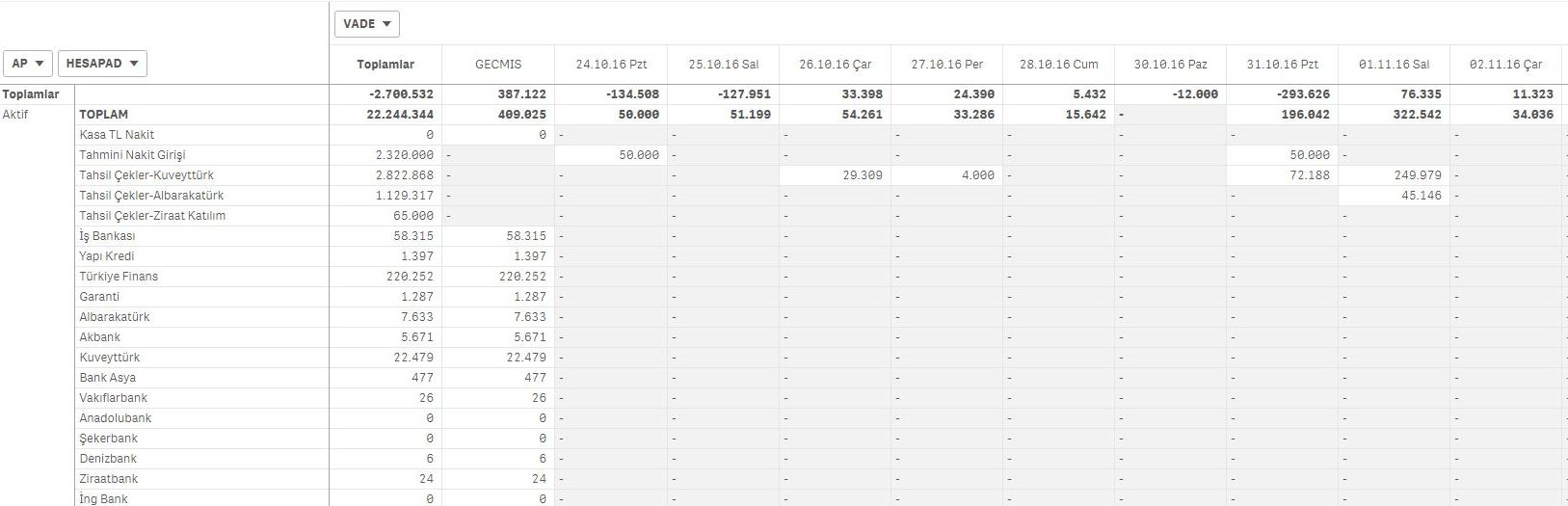New to Qlik Sense

Discussion board where members can get started with Qlik Sense.

New Contributor II

Empty cell in the pivot table is it possible to zero?

Hello there,

Pivot tables dashfields with zero (0) Can we do.

Above(TOTAL SUM(debt),0,RowNo(TOTAL)) + Above(TOTAL SUM(credit),0,RowNo(TOTAL))

+

SUM(amount)1 Solution

Accepted Solutions
Valued Contributor II

Re: Empty cell in the pivot table is it possible to zero?

combination which are not having data in table are displayed as gaps or dashes. You can not show it as zero if you are not having data for that combination.

4 Replies
Valued Contributor II

Re: Empty cell in the pivot table is it possible to zero?

combination which are not having data in table are displayed as gaps or dashes. You can not show it as zero if you are not having data for that combination.

Highlighted
Valued Contributor

Re: Empty cell in the pivot table is it possible to zero?

Hi,

What if you replace the expression by sum({1} 0), does it display 0 values for all the cells? If that is the case then I think you can try something like:

sum({1} 0)

+

alt(

Above(TOTAL SUM(debt),0,RowNo(TOTAL)) + Above(TOTAL SUM(credit),0,RowNo(TOTAL))

+

SUM(amount)

,0)

Valued Contributor